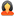Question

#### All Subjects General Questions General General Questions Calculate the slope and intercept of the first order equation

Offered Price \$ 12.00

# Calculate the slope and intercept of the first order equation

Question # 00718288
Subject: General Questions
Topic: General General Questions
Due on: 03/16/2019
Posted On: 03/16/2019 08:47 AM

Rating:
4.1/5Posted ByQuestions:
101096
Tutorials:
101229
Feedback Score:

Question

Question 1

Calculate the slope and intercept of the first order equation y=mx+b  ->  deg_c = m * deg_f + b

Here is the spreadsheet  https://github.com/ml1150258/2019_Spring_CIS_CSC_17c/tree/master/Hmwk/CurveFitLab (Links to an external site.)Links to an external site.

Write a program/project in Netbeans using the same concepts as the  spreadsheet.  You can copy the degrees Fahrenheit column and place in a  file.  Then copy the column with error values included for degree  Celsius.  Calculate the slope and intercept for the data using the  formulas in the spreadsheet.

Question 2

Create abstract classes to inherit, show polymorphic behavior and  operator overloading.  I have written the .h and main.cpp files for  you.  Complete by writing the .cpp class files.

https://github.com/ml1150258/2019_Spring_CIS_CSC_17c/tree/master/Hmwk/Review2_CIS17c_Object_Array

Tutorials for this Question
Available for
\$ 12.00

#### Calculate the slope and intercept of the first order equation

Tutorial # 00718646
Posted On: 03/16/2019 08:48 AM
Posted By:dr.tonyQuestions:
101096
Tutorials:
101229
Feedback Score:
Tutorial Preview … Ca…
Attachments
Calculate_the_slope_and_intercept_of_the_first_order_equation.ZIP (18.96 KB)
* - Additional Paypal / Transaction Handling Fee (3.9% of Tutorial price + \$0.30) applicable